# Case Study for Credit Scorecard Analysis

This example shows how to create a `creditscorecard` object, bin data, display, and plot binned data information. This example also shows how to fit a logistic regression model, obtain a score for the scorecard model, and determine the probabilities of default and validate the credit scorecard model using three different metrics.

### Step 1. Create a creditscorecard object.

Use the `CreditCardData.mat` file to load the `data` (using a dataset from Refaat 2011). If your `data` contains many predictors, you can first use `screenpredictors` (Risk Management Toolbox) to pare down a potentially large set of predictors to a subset that is most predictive of the credit scorecard response variable. You can then use this subset of predictors when creating the `creditscorecard` object. In addition, you can use Threshold Predictors (Risk Management Toolbox) to interactively set credit scorecard predictor thresholds using the output from `screenpredictors` (Risk Management Toolbox).

When creating a `creditscorecard` object, by default, `'ResponseVar'` is set to the last column in the data (`'status'` in this example) and the `'GoodLabel'` to the response value with the highest count (`0` in this example). The syntax for `creditscorecard` indicates that `'CustID'` is the `'IDVar'` to remove from the list of predictors. Also, while not demonstrated in this example, when creating a `creditscorecard` object using `creditscorecard`, you can use the optional name-value pair argument `'WeightsVar'` to specify observation (sample) weights or `'BinMissingData'` to bin missing data.

```load CreditCardData head(data)```
``` CustID CustAge TmAtAddress ResStatus EmpStatus CustIncome TmWBank OtherCC AMBalance UtilRate status ______ _______ ___________ __________ _________ __________ _______ _______ _________ ________ ______ 1 53 62 Tenant Unknown 50000 55 Yes 1055.9 0.22 0 2 61 22 Home Owner Employed 52000 25 Yes 1161.6 0.24 0 3 47 30 Tenant Employed 37000 61 No 877.23 0.29 0 4 50 75 Home Owner Employed 53000 20 Yes 157.37 0.08 0 5 68 56 Home Owner Employed 53000 14 Yes 561.84 0.11 0 6 65 13 Home Owner Employed 48000 59 Yes 968.18 0.15 0 7 34 32 Home Owner Unknown 32000 26 Yes 717.82 0.02 1 8 50 57 Other Employed 51000 33 No 3041.2 0.13 0 ```

The variables in `CreditCardData` are customer ID, customer age, time at current address, residential status, employment status, customer income, time with bank, other credit card, average monthly balance, utilization rate, and the default status (response).

`sc = creditscorecard(data,'IDVar','CustID')`
```sc = creditscorecard with properties: GoodLabel: 0 ResponseVar: 'status' WeightsVar: '' VarNames: {'CustID' 'CustAge' 'TmAtAddress' 'ResStatus' 'EmpStatus' 'CustIncome' 'TmWBank' 'OtherCC' 'AMBalance' 'UtilRate' 'status'} NumericPredictors: {'CustAge' 'TmAtAddress' 'CustIncome' 'TmWBank' 'AMBalance' 'UtilRate'} CategoricalPredictors: {'ResStatus' 'EmpStatus' 'OtherCC'} BinMissingData: 0 IDVar: 'CustID' PredictorVars: {'CustAge' 'TmAtAddress' 'ResStatus' 'EmpStatus' 'CustIncome' 'TmWBank' 'OtherCC' 'AMBalance' 'UtilRate'} Data: [1200x11 table] ```

Perform some initial data exploration. Inquire about predictor statistics for the categorical variable `'ResStatus'` and plot the bin information for `'ResStatus'`.

`bininfo(sc,'ResStatus')`
```ans=4×6 table Bin Good Bad Odds WOE InfoValue ______________ ____ ___ ______ _________ _________ {'Home Owner'} 365 177 2.0621 0.019329 0.0001682 {'Tenant' } 307 167 1.8383 -0.095564 0.0036638 {'Other' } 131 53 2.4717 0.20049 0.0059418 {'Totals' } 803 397 2.0227 NaN 0.0097738 ```
`plotbins(sc,'ResStatus')`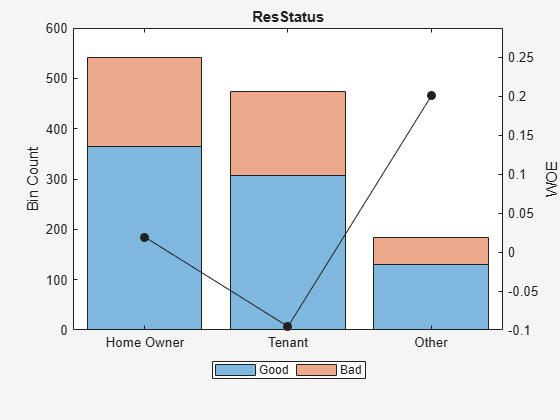This bin information contains the frequencies of “Good” and “Bad,” and bin statistics. Avoid having bins with frequencies of zero because they lead to infinite or undefined (`NaN`) statistics. Use the `modifybins` or `autobinning` functions to bin the data accordingly.

For numeric data, a common first step is "fine classing." This means binning the data into several bins, defined with a regular grid. To illustrate this point, use the predictor `'CustIncome'`.

```cp = 20000:5000:60000; sc = modifybins(sc,'CustIncome','CutPoints',cp); bininfo(sc,'CustIncome')```
```ans=11×6 table Bin Good Bad Odds WOE InfoValue _________________ ____ ___ _______ _________ __________ {'[-Inf,20000)' } 3 5 0.6 -1.2152 0.010765 {'[20000,25000)'} 23 16 1.4375 -0.34151 0.0039819 {'[25000,30000)'} 38 47 0.80851 -0.91698 0.065166 {'[30000,35000)'} 131 75 1.7467 -0.14671 0.003782 {'[35000,40000)'} 193 98 1.9694 -0.026696 0.00017359 {'[40000,45000)'} 173 76 2.2763 0.11814 0.0028361 {'[45000,50000)'} 131 47 2.7872 0.32063 0.014348 {'[50000,55000)'} 82 24 3.4167 0.52425 0.021842 {'[55000,60000)'} 21 8 2.625 0.26066 0.0015642 {'[60000,Inf]' } 8 1 8 1.375 0.010235 {'Totals' } 803 397 2.0227 NaN 0.13469 ```
`plotbins(sc,'CustIncome')`### Step 2a. Automatically bin the data.

Use the `autobinning` function to perform automatic binning for every predictor variable, using the default `'Monotone'` algorithm with default algorithm options.

`sc = autobinning(sc);`

After the automatic binning step, every predictor bin must be reviewed using the `bininfo` and `plotbins` functions and fine-tuned. A monotonic, ideally linear trend in the Weight of Evidence (WOE) is desirable for credit scorecards because this translates into linear points for a given predictor. The WOE trends can be visualized using `plotbins`.

```Predictor =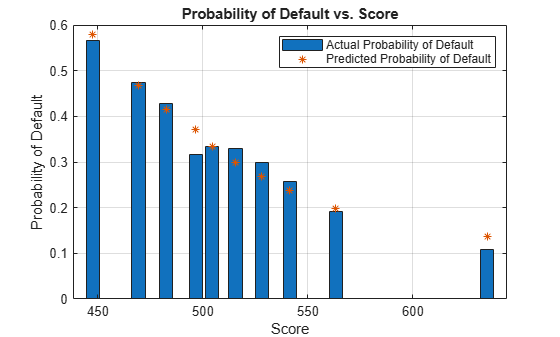'ResStatus'; plotbins(sc,Predictor)```Unlike the initial plot of `'ResStatus'` when the scorecard was created, the new plot for `'ResStatus'` shows an increasing WOE trend. This is because the `autobinning` function, by default, sorts the order of the categories by increasing odds.

These plots show that the `'Monotone'` algorithm does a good job finding monotone WOE trends for this dataset. To complete the binning process, it is necessary to make only a few manual adjustments for some predictors using the `modifybins` function.

### Step 2b. Fine-tune the bins using manual binning.

Common steps to manually modify bins are:

For example, based on the plot for `'CustAge'` in Step 2a, bins number 1 and 2 have similar WOE's as do bins number 5 and 6. To merge these bins using the steps outlined above:

```Predictor ='CustAge'; [bi,cp] = bininfo(sc,Predictor)```
```bi=8×6 table Bin Good Bad Odds WOE InfoValue _____________ ____ ___ ______ _________ _________ {'[-Inf,33)'} 70 53 1.3208 -0.42622 0.019746 {'[33,37)' } 64 47 1.3617 -0.39568 0.015308 {'[37,40)' } 73 47 1.5532 -0.26411 0.0072573 {'[40,46)' } 174 94 1.8511 -0.088658 0.001781 {'[46,48)' } 61 25 2.44 0.18758 0.0024372 {'[48,58)' } 263 105 2.5048 0.21378 0.013476 {'[58,Inf]' } 98 26 3.7692 0.62245 0.0352 {'Totals' } 803 397 2.0227 NaN 0.095205 ```
```cp = 6×1 33 37 40 46 48 58 ```
```cp([1 5]) = []; % To merge bins 1 and 2, and bins 5 and 6 sc = modifybins(sc,'CustAge','CutPoints',cp); plotbins(sc,'CustAge')```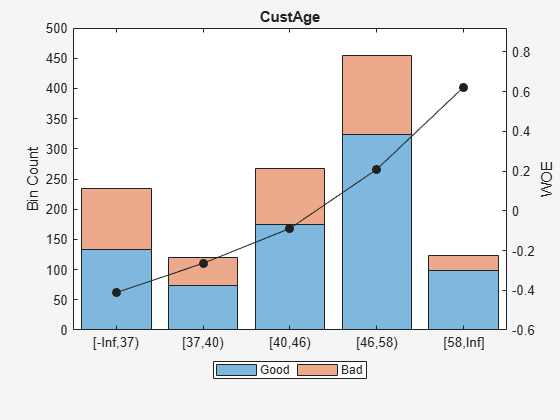For `'CustIncome'`, based on the plot above, it is best to merge bins 3, 4 and 5 because they have similar WOE's. To merge these bins:

```Predictor ='CustIncome'; [bi,cp] = bininfo(sc,Predictor)```
```bi=8×6 table Bin Good Bad Odds WOE InfoValue _________________ ____ ___ _______ _________ __________ {'[-Inf,29000)' } 53 58 0.91379 -0.79457 0.06364 {'[29000,33000)'} 74 49 1.5102 -0.29217 0.0091366 {'[33000,35000)'} 68 36 1.8889 -0.06843 0.00041042 {'[35000,40000)'} 193 98 1.9694 -0.026696 0.00017359 {'[40000,42000)'} 68 34 2 -0.011271 1.0819e-05 {'[42000,47000)'} 164 66 2.4848 0.20579 0.0078175 {'[47000,Inf]' } 183 56 3.2679 0.47972 0.041657 {'Totals' } 803 397 2.0227 NaN 0.12285 ```
```cp = 6×1 29000 33000 35000 40000 42000 47000 ```
```cp([3 4]) = []; % To merge bins 3, 4, and 5 sc = modifybins(sc,'CustIncome','CutPoints',cp); plotbins(sc,'CustIncome')```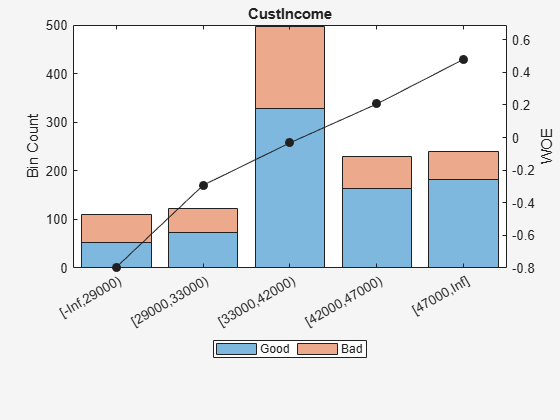For `'TmWBank'`, based on the plot above, it is best to merge bins 2 and 3 because they have similar WOE's. To merge these bins:

```Predictor =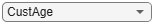'TmWBank'; [bi,cp] = bininfo(sc,Predictor)```
```bi=6×6 table Bin Good Bad Odds WOE InfoValue _____________ ____ ___ ______ ________ _________ {'[-Inf,12)'} 141 90 1.5667 -0.25547 0.013057 {'[12,23)' } 165 93 1.7742 -0.13107 0.0037719 {'[23,45)' } 224 125 1.792 -0.12109 0.0043479 {'[45,71)' } 177 67 2.6418 0.26704 0.013795 {'[71,Inf]' } 96 22 4.3636 0.76889 0.049313 {'Totals' } 803 397 2.0227 NaN 0.084284 ```
```cp = 4×1 12 23 45 71 ```
```cp(2) = []; % To merge bins 2 and 3 sc = modifybins(sc,'TmWBank','CutPoints',cp); plotbins(sc,'TmWBank')```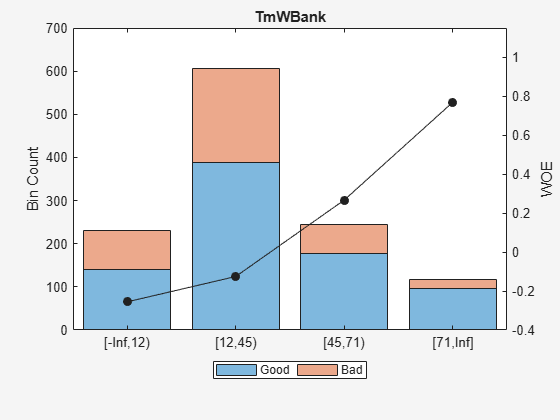For `'AMBalance'`, based on the plot above, it is best to merge bins 2 and 3 because they have similar WOE's. To merge these bins:

```Predictor ='AMBalance'; [bi,cp] = bininfo(sc,Predictor)```
```bi=5×6 table Bin Good Bad Odds WOE InfoValue _____________________ ____ ___ ______ ________ _________ {'[-Inf,558.88)' } 346 134 2.5821 0.24418 0.022795 {'[558.88,1254.28)' } 309 171 1.807 -0.11274 0.0051774 {'[1254.28,1597.44)'} 76 44 1.7273 -0.15787 0.0025554 {'[1597.44,Inf]' } 72 48 1.5 -0.29895 0.0093402 {'Totals' } 803 397 2.0227 NaN 0.039868 ```
```cp = 3×1 103 × 0.5589 1.2543 1.5974 ```
```cp(2) = []; % To merge bins 2 and 3 sc = modifybins(sc,'AMBalance','CutPoints',cp); plotbins(sc,'AMBalance')```Now that the binning fine-tuning is completed, the bins for all predictors have close-to-linear WOE trends.

### Step 3. Fit a logistic regression model.

The `fitmodel` function fits a logistic regression model to the WOE data. `fitmodel` internally bins the training data, transforms it into WOE values, maps the response variable so that `'Good'` is `1`, and fits a linear logistic regression model. By default, `fitmodel` uses a stepwise procedure to determine which predictors should be in the model.

`sc = fitmodel(sc);`
```1. Adding CustIncome, Deviance = 1490.8954, Chi2Stat = 32.545914, PValue = 1.1640961e-08 2. Adding TmWBank, Deviance = 1467.3249, Chi2Stat = 23.570535, PValue = 1.2041739e-06 3. Adding AMBalance, Deviance = 1455.858, Chi2Stat = 11.466846, PValue = 0.00070848829 4. Adding EmpStatus, Deviance = 1447.6148, Chi2Stat = 8.2432677, PValue = 0.0040903428 5. Adding CustAge, Deviance = 1442.06, Chi2Stat = 5.5547849, PValue = 0.018430237 6. Adding ResStatus, Deviance = 1437.9435, Chi2Stat = 4.1164321, PValue = 0.042468555 7. Adding OtherCC, Deviance = 1433.7372, Chi2Stat = 4.2063597, PValue = 0.040272676 Generalized linear regression model: logit(status) ~ 1 + CustAge + ResStatus + EmpStatus + CustIncome + TmWBank + OtherCC + AMBalance Distribution = Binomial Estimated Coefficients: Estimate SE tStat pValue ________ _______ ______ __________ (Intercept) 0.7024 0.064 10.975 5.0407e-28 CustAge 0.61562 0.24783 2.4841 0.012988 ResStatus 1.3776 0.65266 2.1107 0.034799 EmpStatus 0.88592 0.29296 3.024 0.0024946 CustIncome 0.69836 0.21715 3.216 0.0013001 TmWBank 1.106 0.23266 4.7538 1.9958e-06 OtherCC 1.0933 0.52911 2.0662 0.038806 AMBalance 1.0437 0.32292 3.2322 0.0012285 1200 observations, 1192 error degrees of freedom Dispersion: 1 Chi^2-statistic vs. constant model: 89.7, p-value = 1.42e-16 ```

### Step 4. Review and format scorecard points.

After fitting the logistic model, by default the points are unscaled and come directly from the combination of WOE values and model coefficients. The `displaypoints` function summarizes the scorecard points.

```p1 = displaypoints(sc); disp(p1)```
``` Predictors Bin Points ______________ ____________________ _________ {'CustAge' } {'[-Inf,37)' } -0.15314 {'CustAge' } {'[37,40)' } -0.062247 {'CustAge' } {'[40,46)' } 0.045763 {'CustAge' } {'[46,58)' } 0.22888 {'CustAge' } {'[58,Inf]' } 0.48354 {'CustAge' } {'<missing>' } NaN {'ResStatus' } {'Tenant' } -0.031302 {'ResStatus' } {'Home Owner' } 0.12697 {'ResStatus' } {'Other' } 0.37652 {'ResStatus' } {'<missing>' } NaN {'EmpStatus' } {'Unknown' } -0.076369 {'EmpStatus' } {'Employed' } 0.31456 {'EmpStatus' } {'<missing>' } NaN {'CustIncome'} {'[-Inf,29000)' } -0.45455 {'CustIncome'} {'[29000,33000)' } -0.1037 {'CustIncome'} {'[33000,42000)' } 0.077768 {'CustIncome'} {'[42000,47000)' } 0.24406 {'CustIncome'} {'[47000,Inf]' } 0.43536 {'CustIncome'} {'<missing>' } NaN {'TmWBank' } {'[-Inf,12)' } -0.18221 {'TmWBank' } {'[12,45)' } -0.038279 {'TmWBank' } {'[45,71)' } 0.39569 {'TmWBank' } {'[71,Inf]' } 0.95074 {'TmWBank' } {'<missing>' } NaN {'OtherCC' } {'No' } -0.193 {'OtherCC' } {'Yes' } 0.15868 {'OtherCC' } {'<missing>' } NaN {'AMBalance' } {'[-Inf,558.88)' } 0.3552 {'AMBalance' } {'[558.88,1597.44)'} -0.026797 {'AMBalance' } {'[1597.44,Inf]' } -0.21168 {'AMBalance' } {'<missing>' } NaN ```

This is a good time to modify the bin labels, if this is something of interest for cosmetic reasons. To do so, use `modifybins` to change the bin labels.

```sc = modifybins(sc,'CustAge','BinLabels',... {'Up to 36' '37 to 39' '40 to 45' '46 to 57' '58 and up'}); sc = modifybins(sc,'CustIncome','BinLabels',... {'Up to 28999' '29000 to 32999' '33000 to 41999' '42000 to 46999' '47000 and up'}); sc = modifybins(sc,'TmWBank','BinLabels',... {'Up to 11' '12 to 44' '45 to 70' '71 and up'}); sc = modifybins(sc,'AMBalance','BinLabels',... {'Up to 558.87' '558.88 to 1597.43' '1597.44 and up'}); p1 = displaypoints(sc); disp(p1)```
``` Predictors Bin Points ______________ _____________________ _________ {'CustAge' } {'Up to 36' } -0.15314 {'CustAge' } {'37 to 39' } -0.062247 {'CustAge' } {'40 to 45' } 0.045763 {'CustAge' } {'46 to 57' } 0.22888 {'CustAge' } {'58 and up' } 0.48354 {'CustAge' } {'<missing>' } NaN {'ResStatus' } {'Tenant' } -0.031302 {'ResStatus' } {'Home Owner' } 0.12697 {'ResStatus' } {'Other' } 0.37652 {'ResStatus' } {'<missing>' } NaN {'EmpStatus' } {'Unknown' } -0.076369 {'EmpStatus' } {'Employed' } 0.31456 {'EmpStatus' } {'<missing>' } NaN {'CustIncome'} {'Up to 28999' } -0.45455 {'CustIncome'} {'29000 to 32999' } -0.1037 {'CustIncome'} {'33000 to 41999' } 0.077768 {'CustIncome'} {'42000 to 46999' } 0.24406 {'CustIncome'} {'47000 and up' } 0.43536 {'CustIncome'} {'<missing>' } NaN {'TmWBank' } {'Up to 11' } -0.18221 {'TmWBank' } {'12 to 44' } -0.038279 {'TmWBank' } {'45 to 70' } 0.39569 {'TmWBank' } {'71 and up' } 0.95074 {'TmWBank' } {'<missing>' } NaN {'OtherCC' } {'No' } -0.193 {'OtherCC' } {'Yes' } 0.15868 {'OtherCC' } {'<missing>' } NaN {'AMBalance' } {'Up to 558.87' } 0.3552 {'AMBalance' } {'558.88 to 1597.43'} -0.026797 {'AMBalance' } {'1597.44 and up' } -0.21168 {'AMBalance' } {'<missing>' } NaN ```

Points are usually scaled and also often rounded. To do this, use the `formatpoints` function. For example, you can set a target level of points corresponding to a target odds level and also set the required points-to-double-the-odds (PDO).

```TargetPoints = 500; TargetOdds = 2; PDO = 50; % Points to double the odds sc = formatpoints(sc,'PointsOddsAndPDO',[TargetPoints TargetOdds PDO]); p2 = displaypoints(sc); disp(p2)```
``` Predictors Bin Points ______________ _____________________ ______ {'CustAge' } {'Up to 36' } 53.239 {'CustAge' } {'37 to 39' } 59.796 {'CustAge' } {'40 to 45' } 67.587 {'CustAge' } {'46 to 57' } 80.796 {'CustAge' } {'58 and up' } 99.166 {'CustAge' } {'<missing>' } NaN {'ResStatus' } {'Tenant' } 62.028 {'ResStatus' } {'Home Owner' } 73.445 {'ResStatus' } {'Other' } 91.446 {'ResStatus' } {'<missing>' } NaN {'EmpStatus' } {'Unknown' } 58.777 {'EmpStatus' } {'Employed' } 86.976 {'EmpStatus' } {'<missing>' } NaN {'CustIncome'} {'Up to 28999' } 31.497 {'CustIncome'} {'29000 to 32999' } 56.805 {'CustIncome'} {'33000 to 41999' } 69.896 {'CustIncome'} {'42000 to 46999' } 81.891 {'CustIncome'} {'47000 and up' } 95.69 {'CustIncome'} {'<missing>' } NaN {'TmWBank' } {'Up to 11' } 51.142 {'TmWBank' } {'12 to 44' } 61.524 {'TmWBank' } {'45 to 70' } 92.829 {'TmWBank' } {'71 and up' } 132.87 {'TmWBank' } {'<missing>' } NaN {'OtherCC' } {'No' } 50.364 {'OtherCC' } {'Yes' } 75.732 {'OtherCC' } {'<missing>' } NaN {'AMBalance' } {'Up to 558.87' } 89.908 {'AMBalance' } {'558.88 to 1597.43'} 62.353 {'AMBalance' } {'1597.44 and up' } 49.016 {'AMBalance' } {'<missing>' } NaN ```

### Step 5. Score the data.

The `score` function computes the scores for the training data. An optional `data` input can also be passed to `score`, for example, validation data. The points per predictor for each customer are provided as an optional output.

```[Scores,Points] = score(sc); disp(Scores(1:10))```
``` 528.2044 554.8861 505.2406 564.0717 554.8861 586.1904 441.8755 515.8125 524.4553 508.3169 ```
`disp(Points(1:10,:))`
``` CustAge ResStatus EmpStatus CustIncome TmWBank OtherCC AMBalance _______ _________ _________ __________ _______ _______ _________ 80.796 62.028 58.777 95.69 92.829 75.732 62.353 99.166 73.445 86.976 95.69 61.524 75.732 62.353 80.796 62.028 86.976 69.896 92.829 50.364 62.353 80.796 73.445 86.976 95.69 61.524 75.732 89.908 99.166 73.445 86.976 95.69 61.524 75.732 62.353 99.166 73.445 86.976 95.69 92.829 75.732 62.353 53.239 73.445 58.777 56.805 61.524 75.732 62.353 80.796 91.446 86.976 95.69 61.524 50.364 49.016 80.796 62.028 58.777 95.69 61.524 75.732 89.908 80.796 73.445 58.777 95.69 61.524 75.732 62.353 ```

### Step 6. Calculate the probability of default.

To calculate the probability of default, use the `probdefault` function.

`pd = probdefault(sc);`

Define the probability of being “Good” and plot the predicted odds versus the formatted scores. Visually analyze that the target points and target odds match and that the points-to-double-the-odds (PDO) relationship holds.

```ProbGood = 1-pd; PredictedOdds = ProbGood./pd; figure scatter(Scores,PredictedOdds) title('Predicted Odds vs. Score') xlabel('Score') ylabel('Predicted Odds') hold on xLimits = xlim; yLimits = ylim; % Target points and odds plot([TargetPoints TargetPoints],[yLimits(1) TargetOdds],'k:') plot([xLimits(1) TargetPoints],[TargetOdds TargetOdds],'k:') % Target points plus PDO plot([TargetPoints+PDO TargetPoints+PDO],[yLimits(1) 2*TargetOdds],'k:') plot([xLimits(1) TargetPoints+PDO],[2*TargetOdds 2*TargetOdds],'k:') % Target points minus PDO plot([TargetPoints-PDO TargetPoints-PDO],[yLimits(1) TargetOdds/2],'k:') plot([xLimits(1) TargetPoints-PDO],[TargetOdds/2 TargetOdds/2],'k:') hold off```### Step 7. Validate the credit scorecard model using the CAP, ROC, and Kolmogorov-Smirnov statistic

The `creditscorecard` class supports three validation methods, the Cumulative Accuracy Profile (CAP), the Receiver Operating Characteristic (ROC), and the Kolmogorov-Smirnov (KS) statistic. For more information on CAP, ROC, and KS, see Cumulative Accuracy Profile (CAP), Receiver Operating Characteristic (ROC), and Kolmogorov-Smirnov statistic (KS).

`[Stats,T] = validatemodel(sc,'Plot',{'CAP','ROC','KS'});`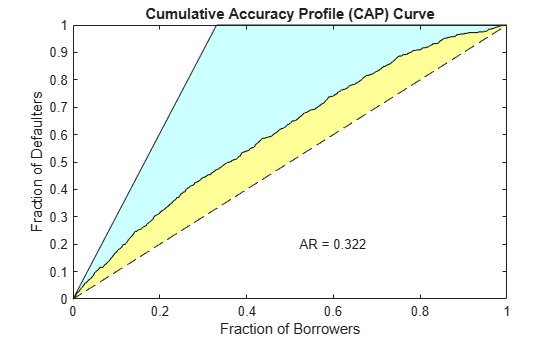`disp(Stats)`
``` Measure Value ________________________ _______ {'Accuracy Ratio' } 0.32225 {'Area under ROC curve'} 0.66113 {'KS statistic' } 0.22324 {'KS score' } 499.18 ```
`disp(T(1:15,:))`
``` Scores ProbDefault TrueBads FalseBads TrueGoods FalseGoods Sensitivity FalseAlarm PctObs ______ ___________ ________ _________ _________ __________ ___________ __________ __________ 369.4 0.7535 0 1 802 397 0 0.0012453 0.00083333 377.86 0.73107 1 1 802 396 0.0025189 0.0012453 0.0016667 379.78 0.7258 2 1 802 395 0.0050378 0.0012453 0.0025 391.81 0.69139 3 1 802 394 0.0075567 0.0012453 0.0033333 394.77 0.68259 3 2 801 394 0.0075567 0.0024907 0.0041667 395.78 0.67954 4 2 801 393 0.010076 0.0024907 0.005 396.95 0.67598 5 2 801 392 0.012594 0.0024907 0.0058333 398.37 0.67167 6 2 801 391 0.015113 0.0024907 0.0066667 401.26 0.66276 7 2 801 390 0.017632 0.0024907 0.0075 403.23 0.65664 8 2 801 389 0.020151 0.0024907 0.0083333 405.09 0.65081 8 3 800 389 0.020151 0.003736 0.0091667 405.15 0.65062 11 5 798 386 0.027708 0.0062267 0.013333 405.37 0.64991 11 6 797 386 0.027708 0.007472 0.014167 406.18 0.64735 12 6 797 385 0.030227 0.007472 0.015 407.14 0.64433 13 6 797 384 0.032746 0.007472 0.015833 ```